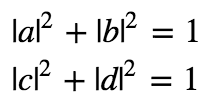# Communication through entanglement

Is it possible to use quantum entanglement to communicate faster than light?

Here’s a suggestion for how we might achieve just such a thing. Two people each possess one qubit of an entangled pair in the state ⟩: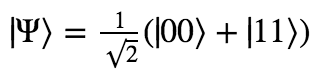The owner of the second qubit then decides whether or not to apply some quantum gate U to their qubit. Immediately following this, the owner of the first qubit measures their qubit.

If the application of U to the second qubit changes the amplitude distribution over the first qubit, then the measurement can be used to communicate a message between the two people instantaneously! Why? Well, initially 0 and 1 are expected with equal probability. But if applying U makes these probabilities unequal, then the observation of a 0 or 1 carries evidence as to whether or not U was applied. (In an extreme case, applying U could make it guaranteed that the qubit would be observed as |0⟩, in which case observation of |1⟩ ensures that U was not applied.)

In this way, somebody could send information across by encoding it in a string of decisions about whether or not to apply U to a shared entangled pair.

It might seem a little strange that doing something to your qubit over here could affect the state of their qubit over there. But this is quantum mechanics, and quantum mechanics is very, very strange. Remember that the two qubits are entangled with one another. We are already guaranteed that what happens to one can affect the state of the other instantaneously – after all, if we measure the second qubit and find it in the state |0⟩, the first qubit’s state is instantaneously “collapsed” into the state |0⟩ as well. (This fact alone cannot be used to communicate messages, because before measuring the second qubit, its owner has no control over which of the two states it will end up in.)

So whether or not our scheme will work cannot be ruled out a priori. We must work out the math for ourselves to see if applying U to qubit 2 can successfully warp the probability distribution of qubit 1, thus sending information between the two instantaneously.

First, we’ll describe our single qubit gate U as a matrix.a, b, c, and d are complex numbers. Can they have any possible values?

No. U must preserve the normalization of states it acts on. In other words, for U to represent a physically possible transformation of a qubit, it cannot transform physically possible states into physically impossible states.

What precise constraints does this entail? It turns out that the following two suffice to ensure the normalization condition:Alright. Now, we have our general description of a single-qubit gate. Of course, the qubit that U is operating on is a part of an entangled pair. It is an irreducible component of a two-qubit system. So we can’t actually describe it as just a single qubit.

Instead, we need to describe the state of both qubits, which, I’ll remind you, looks like: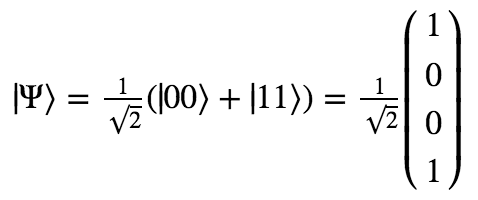A 2×2 matrix can’t operate on a vector with four components. What we need is a two-qubit quantum gate that corresponds to applying U to qubit 2 while leaving qubit 1 alone. “Leaving a qubit alone” is equivalent to applying the identity gate I to it, which just leaves the state unchanged.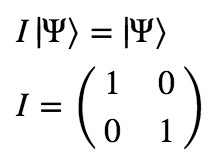So what we really want is the 4×4 matrix that corresponds to applying U to qubit 2 and I to qubit 1. It turns out that we can generate this matrix by simply taking the tensor product of U with I:Alright, now we’re ready to see what happens when we apply this gate to ⟩!This state sure looks different than the state we started with! But is it different enough to have carried some information between the qubits? Let’s now look at the probabilities for measuring qubit 1 in the states 0 and 1: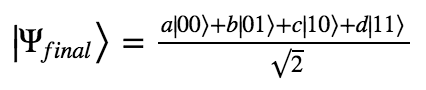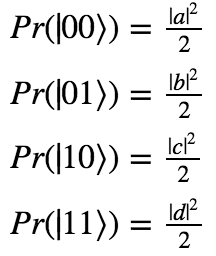Remember the constraints on the possible values of a, b, c, and d we started with?